Next: Searching for More Than Up: Open Questions Previous: Open Questions   Contents

## How Many Iterations are Required

Our initial state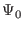= (1/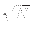, 1/,..., 1/), is attained by performing the Walsh-Hadamard transformation on the register in the zero state.

Let (k, l ) denote denote the state of our vector, where k is the amplitude of the marked state, and l is the amplitude of each of the remaining (N - 1) states. It is the case in Grover's algorithm that the unmarked states always have the same amplitude, so we can use this shorthand.

After the first application of the Walsh-Hadamard operator to place us in an equal superposition of states let us say we are in state= (k0, l0).

From theorem 1 we see the j'th iteration will produce the state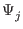= (kj, lj), where k0 = l0 = 1/, further:

kj+1 =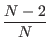kj +lj

lj+1 =lj -kj

With a little work on the recurrence relation we an solve for closed form solutions of k and j. Let the angle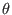be defined so that sin2= 1/N. It can be shown through mathematical induction that:

kj+1 = sin((2j+1)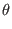)

lj+1 =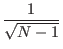cos((2j + 1))

[BBHT96]

We are interested in the number of iterations for k to have near unit probability. Evidently, we will find the register to be in the target state with unit probability when (2m + 1)=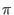/2, or when m = (-2)/4. We can only perform an integer number of iterations, but the probability of failure is less than 1/N if we iterate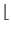/4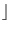times, which is very close to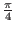when N is large ( 1/= sin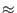). [BBHT96] For the 50 percent probability called for by Grover's algorithm we need only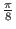iterations. [BBHT96]Next: Searching for More Than Up: Open Questions Previous: Open Questions   Contents
Matthew Hayward - Quantum Computing and Grover's Algorithm GitHub Repository